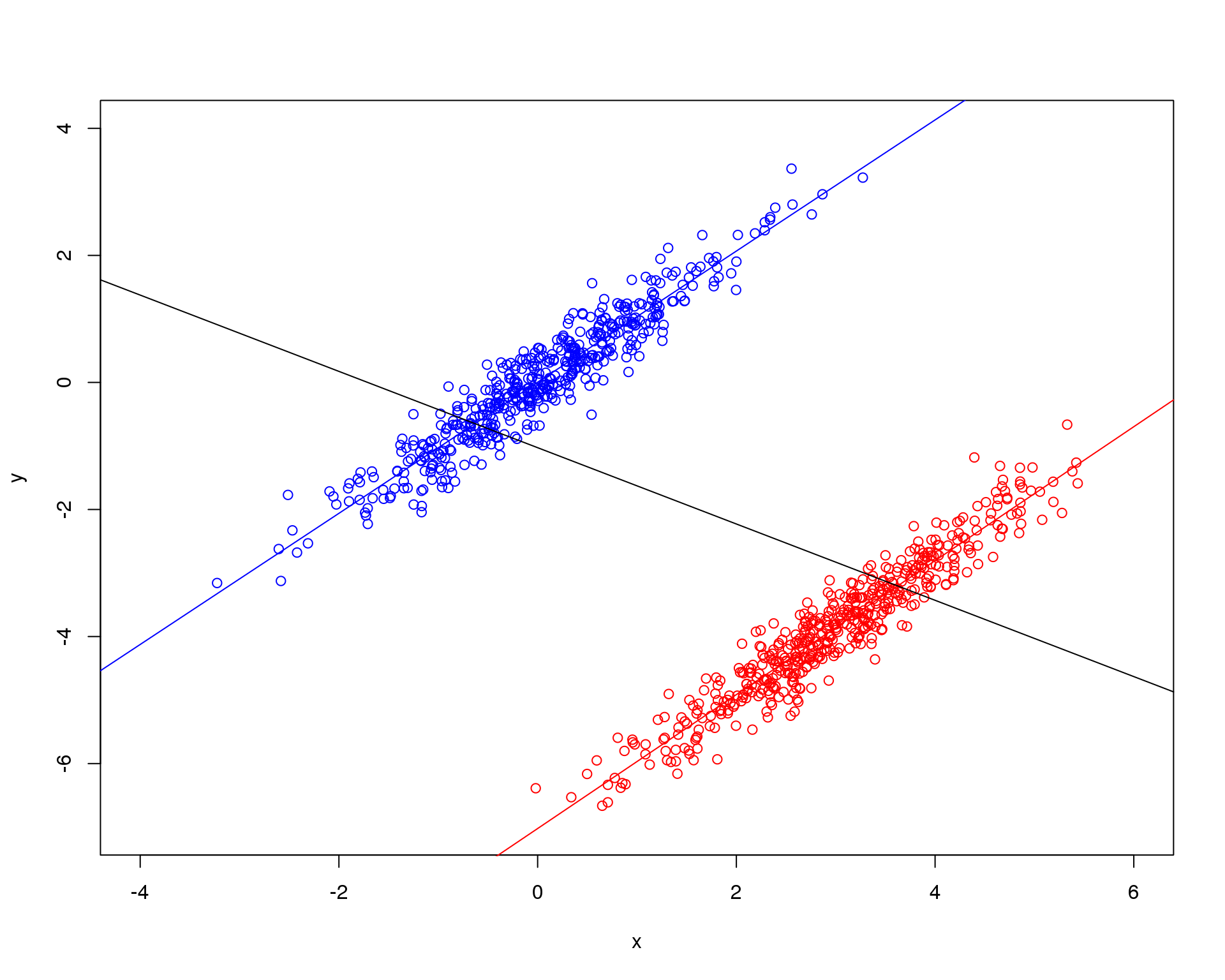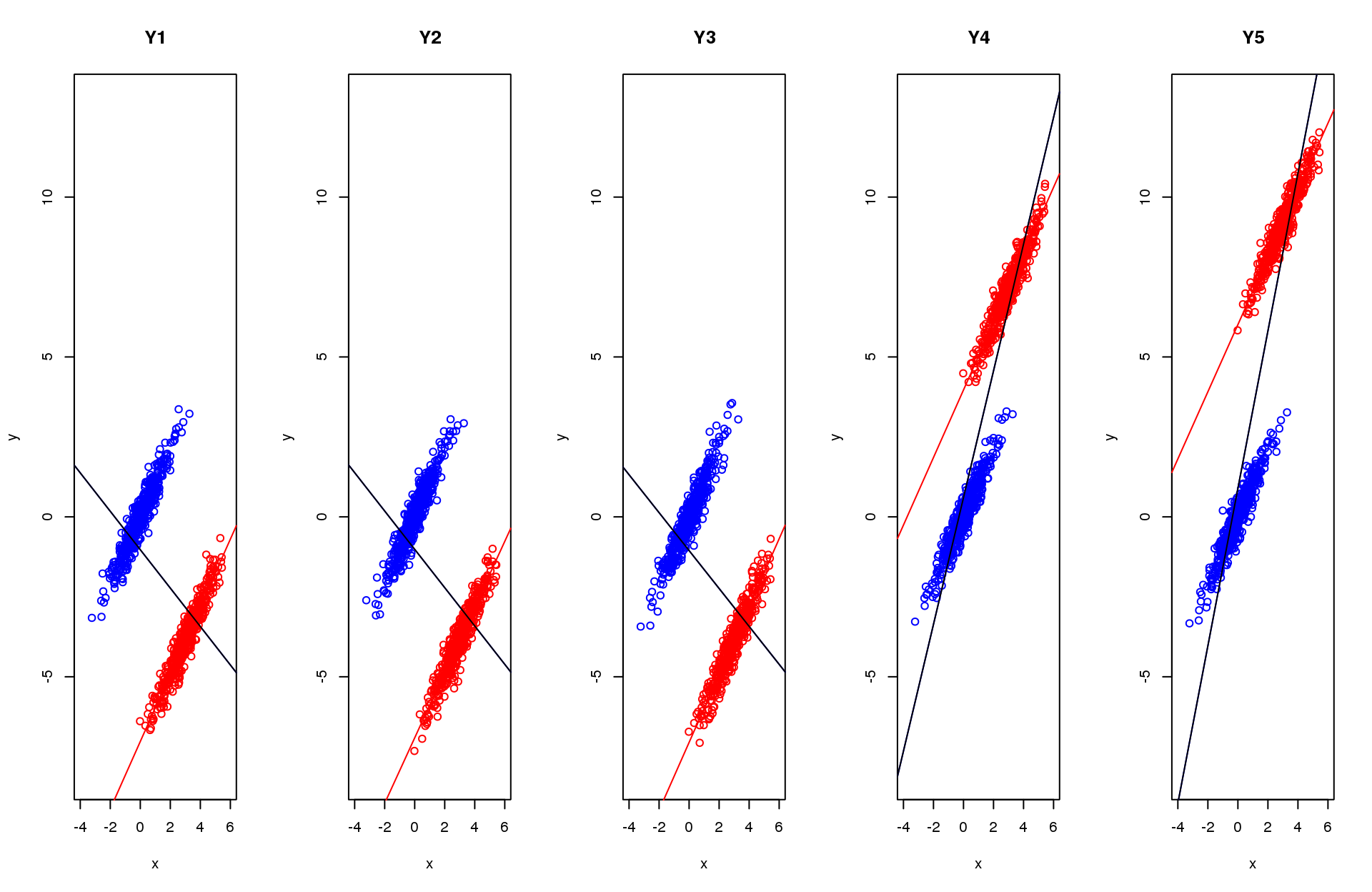## Document format and generation

This is an R Markdown document. Markdown is a simple formatting syntax for authoring HTML, PDF, and MS Word documents. For more details on using R Markdown see http://rmarkdown.rstudio.com.

## General introduction

This started as a way of reassuring myself that my anxieties about an exploratory factor analysis (EFA) of data from the COPES scale where the data came from 21 different therapeutic communities (TCs) and from staff and non-staff members of the those communities and included some repeat data as well as one off data might find an EFA picture of the psychometric structure of the replies that relected more the differences between the units and perhaps the staff/non-staff difference and even perhaps the initial/later issue. It’s turned into a bit of an experiment in using the latest “weaving” capabilities within Rmarkdown and Rstudio to mix text and code and generate HTML, PDF or Word documents.

## Statistical issue

There’s a simple bivariate equivalent of this EFA issue: that the correlation or regression beween two variables may be the same in two groups but the groups so different in mean values on the two variables that the overall correlation or regression between the two in the pooled dataset is quite different from that in each group.

options(width=120)
nGrps <- 2
nInGrp <- 500
grp2XInc <- 3
grp2YDec <- 7
ratio <- 3
slope <- 1.05
grp1x <- rnorm(nInGrp)
grp1err <- rnorm(nInGrp)/ratio
grp2x <- rnorm(nInGrp) + grp2XInc
grp2err <- rnorm(nInGrp)/ratio
grp1y1 <- slope*grp1x + grp1err
grp2y <- slope*grp2x + grp2err - grp2YDec
xMeanDiff <- mean(grp2x) - mean(grp1x)
yMeanDiff <- mean(grp2y) - mean(grp1y1)
grp1Pearson <- cor(grp1x,grp1y1)
grp2Pearson <- cor(grp1x,grp1y1)
x <- c(grp1x,grp2x)
y <- c(grp1y1,grp2y)
allPearson <- cor(x,y)
xmin <- floor(min(x))
xmax <- ceiling(max(x))
ymin <- floor(min(y))
ymax <- ceiling(max(y))
par(col=1)
plot(grp1x,grp1y1,
xlim=c(xmin,xmax),
ylim=c(ymin,ymax),
xlab="x",
ylab="y",
type="n")
par(col="blue")
points(grp1x,grp1y1,
xlim=c(-4,5),
ylim=c(-15,4))
abline(lm(grp1y1 ~ grp1x))
par(col="red")
points(grp2x,grp2y,
xlim=c(-4,5),
ylim=c(-15,4))
abline(lm(grp2y ~ grp2x))
par(col=1)
abline(lm(y ~ x))So that used R’s pseudorandom number generator to generate Gaussian x and y variables in 2 groups each with 500 data points and with exactly the same relationship between the x and y variables but with a substantial mean shift on each variable between the groups. The population value difference is a x increase of 3 and a y decrease of 7. The sample mean x difference is 2.97 and the y difference is -3.9. The correlation between x and y in group 1 is 0.95 and that in group 2 is 0.95 but the overall Pearson correlation between x and y pooled across the two groups is very different at -0.48.

It’s pretty easy to see how this happens from the plot.

## OK, let’s go to the simple multivariate case

This is really very similar to the above but this time I have generated four more y variables within each group, still keeping the x/y relationship the same for all y and within both groups. However, I have arranged things so that the group differences for Y1, Y2 and Y3 are the same (and the same as they were in the bivariate example above) but the group differences for Y4 and Y5 are rather different.

### group 1
grp1err2 <- rnorm(nInGrp)/ratio
grp1y2 <- slope*grp1x + grp1err2
grp1err3 <- rnorm(nInGrp)/ratio
grp1y3 <- slope*grp1x + grp1err3
grp1err4 <- rnorm(nInGrp)/ratio
grp1y4 <- slope*grp1x + grp1err4
grp1err5 <- rnorm(nInGrp)/ratio
grp1y5 <- slope*grp1x + grp1err5
### group 2
grp2err2 <- rnorm(nInGrp)/ratio
grp2YDec2 <- grp2YDec
grp2y2 <- slope*grp2x + grp2err2 - grp2YDec2
grp2err3 <- rnorm(nInGrp)/ratio
grp2YDec3 <- grp2YDec
grp2y3 <- slope*grp2x + grp2err3 - grp2YDec3
grp2err4 <- rnorm(nInGrp)/ratio
grp2YDec4 <- -4
grp2y4 <- slope*grp2x + grp2err4 - grp2YDec4
grp2err5 <- rnorm(nInGrp)/ratio
grp2YDec5 <- -6
grp2y5 <- slope*grp2x + grp2err5 - grp2YDec5

grp1yAll <- cbind(grp1y1,grp1y2,grp1y3,grp1y4,grp1y5)
grp2yAll <- cbind(grp2y,grp2y2,grp2y3,grp2y4,grp2y5)
yAll <- rbind(grp1yAll,grp2yAll)

The plots below show the rather different overall x/y correlations this gives for Y4 and Y5 from Y1, Y2 and Y3.

par(mfrow=c(1,5))
par(col=1)
combPlotFun <- function(x,y,title,xmin,xmax,ymin,ymax) {
par(col=1)
nGrp <- length(x)/2
grp1x <- x[1:nGrp]
grp1y1 <- y[1:nGrp]
grp2x <- x[nGrp+1:length(x)]
grp2y <- y[nGrp+1:length(y)]
plot(x,y,
xlim=c(xmin,xmax),
ylim=c(ymin,ymax),
xlab="x",
ylab="y",
main=title,
type="n")
par(col="blue")
points(grp1x,grp1y1)
abline(lm(y ~ x))
par(col="red")
points(grp2x,grp2y,
xlim=c(-4,5),
ylim=c(-15,4))
abline(lm(grp2y ~ grp2x))
par(col=1)
abline(lm(y ~ x))
}
ymin <- floor(min(yAll))
ymax <- ceiling(max(yAll))
for (i in 1:5) {
combPlotFun(x,yAll[,i],paste("Y",i,sep=""),xmin,xmax,ymin,ymax)
}I’m only going to use these five y variables in the EFA. I’m going to speed things up and keep them simple by actually doing a principal component analysis (PCA). There’s ample evidence that the differences beween PCA loading matrices and those for “true EFAs” of whatever type will be small.

Their correlation matrix for the five y variables within group 1 is:

##        grp1y1 grp1y2 grp1y3 grp1y4 grp1y5
## grp1y1   1.00   0.91   0.90   0.90    0.9
## grp1y2   0.91   1.00   0.89   0.91    0.9
## grp1y3   0.90   0.89   1.00   0.90    0.9
## grp1y4   0.90   0.91   0.90   1.00    0.9
## grp1y5   0.90   0.90   0.90   0.90    1.0

and within group 2 it is:

##        grp2y grp2y2 grp2y3 grp2y4 grp2y5
## grp2y   1.00   0.91   0.90   0.91   0.91
## grp2y2  0.91   1.00   0.90   0.90   0.91
## grp2y3  0.90   0.90   1.00   0.90   0.91
## grp2y4  0.91   0.90   0.90   1.00   0.92
## grp2y5  0.91   0.91   0.91   0.92   1.00

But the correlation for the pooled data is rather different:

##        grp1y1 grp1y2 grp1y3 grp1y4 grp1y5
## grp1y1   1.00   0.98   0.98  -0.71  -0.75
## grp1y2   0.98   1.00   0.98  -0.71  -0.75
## grp1y3   0.98   0.98   1.00  -0.71  -0.75
## grp1y4  -0.71  -0.71  -0.71   1.00   0.99
## grp1y5  -0.75  -0.75  -0.75   0.99   1.00

Of course, this produces very different PCA results for the pooled data from the analyses for each group separately. This shows the scree plots for the two groups separately then for the compbined data.It’s easy to see that the pooled data has a scree plot supporting a two component solution whereas the plots for the within group analyses show a very clear unicomponent solution is fine to capture the systematic covariance in the data.

This is nice and clear in the loadings: here for group 1.

grp1PCA$rotation ## PC1 PC2 PC3 PC4 PC5 ## grp1y1 0.4427 -0.33545 0.03453 -0.7632 -0.3283 ## grp1y2 0.4505 -0.48864 -0.10811 0.1608 0.7216 ## grp1y3 0.4531 0.79826 -0.18226 -0.2161 0.2785 ## grp1y4 0.4404 -0.06835 -0.55573 0.4805 -0.5116 ## grp1y5 0.4492 0.08251 0.80316 0.3379 -0.1795 And here for the pooled data. (I’ve omitted group 2 as it’s essentially identical to group 1.) allPCA$rotation
##            PC1     PC2      PC3      PC4      PC5
## grp1y1 -0.2814 -0.5017  0.37308 -0.33312 -0.64725
## grp1y2 -0.2802 -0.4992  0.43402  0.15880  0.67725
## grp1y3 -0.2802 -0.5084 -0.81129  0.06805  0.01327
## grp1y4  0.5376 -0.3577  0.09356  0.70904 -0.26748
## grp1y5  0.6890 -0.3356 -0.07408 -0.59703  0.22513

Varimax rotation of first two components from the pooled data:

varimax(allPCA$rotation[,1:2]) ##$loadings
##
##        PC1    PC2
## grp1y1        -0.575
## grp1y2        -0.572
## grp1y3        -0.580
## grp1y4  0.645
## grp1y5  0.764
##
##                PC1 PC2
## [2,] -0.5028 0.8644